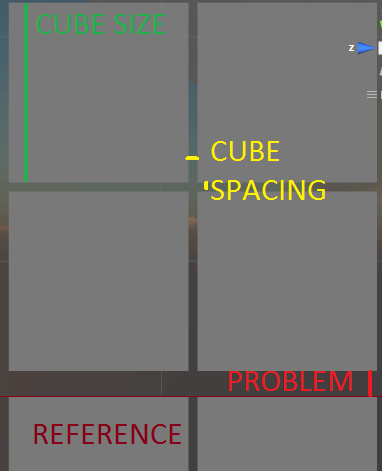# How to move several objects "y" units during a fixed time

I have a selection of transforms that I need to move a certain amount of units up during a fixed time. This is my corotine.

``````float timer = 0f;
while (timer < riseTime) {
foreach (Transform trans in sel.list) {
pos = trans.position;
pos.y = pos.y + (sel.y *(cubeSize+cubeSpacing) * Time.deltaTime) / riseTime;
trans.position = pos;
}
timer+= Time.deltaTime;
yield return null;
}
``````

The distance between the last object and my visual reference is never the same. I can’t fix it and I would really appreciate any help.

I have done it beautifully with Vector3.Lerp but I need to store the initial positions for all the cubes in an array and that just doesn’t sound good to me.

this works, not sure if its the kind of thing you are after though.

``````using UnityEngine;
using System.Collections;

public class moveDir : MonoBehaviour {

// Use this for initialization

float timeToMove = 1f;
float currentTime;
GameObject[] cubeList;
bool move = true;

void Start () {
cubeList = GameObject.FindGameObjectsWithTag("cube");
currentTime = Time.time + timeToMove;
}

// Update is called once per frame
void Update () {
if(move)
{
moveCubes(timeToMove);
}
if(!move)
{
currentTime = Time.time + timeToMove;
}
if(Input.GetKeyDown(KeyCode.A))
{
move = !move;
}
}

void moveCubes(float delayTime)
{

if(Time.time < currentTime)
{

foreach(GameObject cube in cubeList)
{
cube.transform.position += new Vector3(0,1,0)*Time.deltaTime;
}
}
}

}
``````